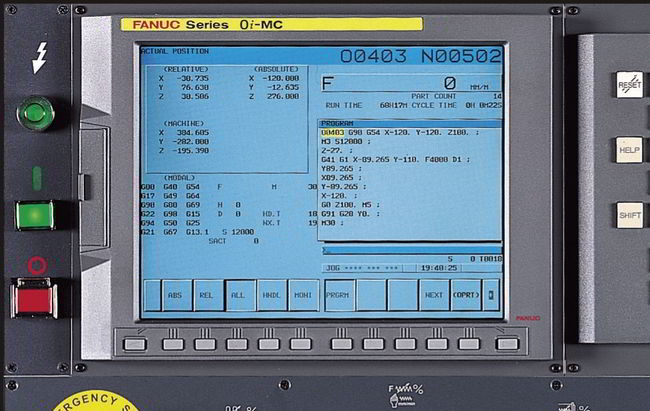# Fanuc Decimal Point Programming Introduction / Parameters

## Fanuc Decimal Point Programming

### Summary

On some cnc controls while programming cnc programmers have to put decimal point at the end of most numeric values e.g. X100.0 Y10.0
While on most of the cnc controls it is not necessary to put a decimal point at the end of a numeric value like X100 Y10
On Fanuc cnc controls this behavior is parameter (No.3401#0 or No.2400#0) dependent.### Description

Numerical values can be entered with a decimal point. A decimal point can be used when entering a distance, time, or speed. Decimal points can be specified with the following addresses:
Milling
X, Y, Z, U, V, W, A, B, C, I, J, K, Q, R, F
Turning
X, Y, Z, U, V, W, A, B, C, I, J, K, R, F

## Decimal Point Programming Types

There are two types of decimal point notation:

• Calculator-type notation
• Standard notation

### Calculator-type Notation

When calculator-type decimal notation is used, a value without decimal point is considered to be specified in millimeters inch,or deg.

### Standard Notation

When standard decimal notation is used, such a value is considered to be specified in least input increments. Select either calculator-type or standard decimal notation by using the parameter DPI (No.3401#0 or No.2400#0).
Values can be specified both with and without decimal point in a single program.

## Examples

Program commandPocket calculator type decimal point programmingStandard type decimal point programming
X1000
Command value without decimal point
1000mm
Unit :mm
1mm
Unit : Least input increment (0.001mm)
X1000.0
Command value with decimal point
1000mm
Unit :mm
1000mm
Unit :mm

## Fanuc Parameter 3401

Fanuc parameter 3401 works on

• Fanuc Series 30i/31i/32i
• Fanuc Series 0i
• Fanuc 16i18i21i
• Fanuc 16/18/21
#7#6#5#4#3#2#1#0
DPI

Input type Parameter input
Data type Bit path
#0 DPI When a decimal point is omitted in an address that can include a decimal point

• 0: The least input increment is assumed. (Normal decimal point input)
• 1: The unit of mm, inches, degree, or second is assumed. (Pocket calculator type decimal point input)

## Fanuc Parameter 2400

Fanuc parameter 2400 works on

• Fanuc 15
#7#6#5#4#3#2#1#0
DPI

Parameter input
Data type : Bit
DPI A value with the decimal point is entered

• 0 : By the conventional method.
• 1 : In fixed–point notation.